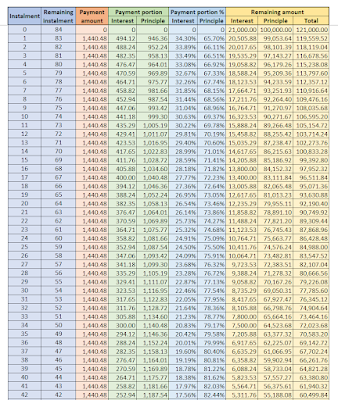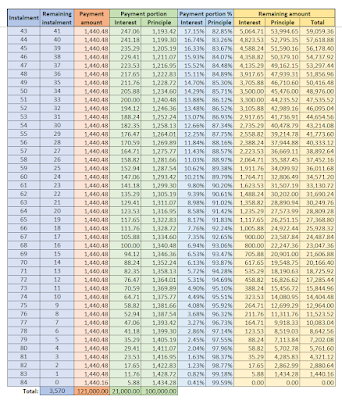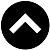-->

Type something and hit enter

# How much can you save from loan early settlement?

How much can you save from loan early settlement?

Typically, there are 2 types of loans. One is fixed interest rate type, and another is variable interest rate type.

Some examples of fixed interest rate loan are: car loan, personal loan, credit card loan, study loan, business loan, etc.

Since the interest rate is fixed throughout the loan tenure, you can calculate its total interest using the simple interest formula of P x R% x T. The total loan repayment amount will then be principal plus total interest. The installment amount will be total repayment amount divided by no. of installments.

Variable interest rate loan has its interest calculated daily (daily rest), monthly (monthly rest), or other predetermined interval period. Property loan is normally of this type. settlements

For this type of loan, since the interest rate in the future is unpredictable, the actual interest saved from early settlement is also unpredictable until the time has reached its original repayment end date. If we fix the last interest rate before the early settlement made for the rest of the original tenure, then the interest saved can be estimated using the simple interest formula above. Just substitute P with the early settlement amount will do.

The rest of this article will focus on how much we can save from early settlement of fixed interest rate loan.

The interest saved is usually called interest rebate, and is calculated according to the rule of 78. For Islamic loans, it is calculated according to the formula of Ibra'. Basically, both formulae are identical.

As an example, let's say a 7 years loan with principal 100k, 3% interest per annum, if we fully settle it 3 years after the borrowing, how much interest rebate can we get back?

Principal = 100k

Interest = 100k x 3% x 7 = 21k

7 years total repayment = 100k + 21k = 121k

Monthly installment = 121k/84 = 1440.476, rounded up to 1440.48

Since there is a round up of 0.004 for every installment, the last installment will then be 1440.16 only.

The sequential sum of all installment no. = 1+2+3+...+82+83+84 = (84)(84+1)/2 = 3570

According to rule of 78:

Interest for installment no. 1 = 21k x 84/3570

Interest for installment no. 2 = 21k x 83/3570

Interest for installment no. 3 = 21k x 82/3570

and so on...

Putting all the above information into an Excel worksheet, we can produce the following...and from the table above, we can produce the following graphs for better visualization of the interest portion:

Back to our question, if we make an early settlement at the 36th installment, how much interest rebate can we get?

The interest rebate will be the sum of interest portion of the 37th installment until the last 84th installment.

There is a formula to quickly calculate this interest rebate:

Interest rebate = n(n+1)/N(N+1) x I

where:

N is the overall no. of installments

n is the no. of remaining installments

I is the overall interest amount = P x R% x T

Many financial institutions require an early notice period of at least 3 months, else a penalty will be imposed based on the interest amount of the notice shortcoming installments.

Some financial institutions also charge a fee, such as processing free, or early settlement fee, for early settlement.

By including these factors, our final formula will be:

Interest rebate = (n-t)(n-t+1)/N(N+1) x I - C

where:

t is the shortcoming of notice period

C is the related charge for early settlement

Last but not least, looking at our example above, we can observe that the interest is nearly fully paid off during the last 1.5 years of the loan tenure. As such, the interest rebate available in that time range is very minimal, and you can't save much for making early settlement at the final stage of the loan tenure.

http://voyager8.blogspot.com/2022/07/how-much-can-you-save-from-loan-early.html